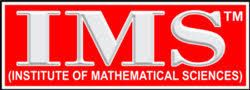# CSAT Test Series (Full Syllabus) Paper – Set 2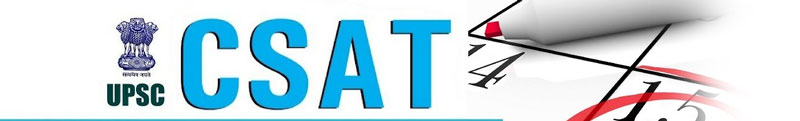Ques.No-1 Directions (17–20): Study the following graph carefully and answer the questions given below:

Percentage of Different Types of Employees in a Company in two successive years Total No. of Employees in 1997 & 1998 is 42,980 and 48,640 respectively.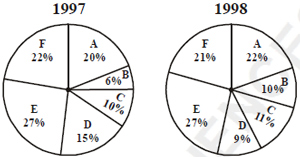The number of A type employees in 1998 was approximately what percentage of A type employees in 1997?

1. 115
2. 140
3. 125
4. 130

Ques.No-2: If there were 5,000 D type employees in 1998, what would have been its approximate percentage in the company?

1. 8
2. 12
3. 14
4. 10

Ques.No-3: In the case of which of the following types of employees was there maximum change in the number from1997 to 1998 ?

1. B
2. D
3. C
4. A

Ques.No-4: Approximately, what was the difference in the number of B type employees between 1997 and 1998 ?

1. 2285
2. 2325
3. 2085
4. 2620

Ques.No-5: The total number of which of the following pairs of types of employees in 1997 was approximately equal to A type employees in 1998 ?

1. B and C
2. A and C
3. D and E
4. C and D

Ques.No-6: An athlete is running in a circular track whose radius is 77m. How many rounds will he complete to run a race of 4840m?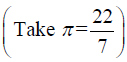1. 8
2. 12
3. 9
4. 10

Ques.No-7: One-third of the girls and one-half of the boys of a college participate in a social service project. If the total number of girls is half of that of boys in the college, what fractional part of the total student population participates in the project?

a.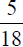b.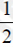c.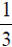d. None of these

Ques.No-8: In how many different ways can all of 5 identical balls be placed in the cells shown above such that each row contains at least1ball ?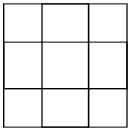1. 64
2. 81
3. 84
4. 108

Select the numbered figure that will replace the question mark (?) in figure (D) to complete the given patterna.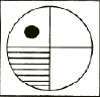b.c.d.Answerkeys:Ques-1 : (c), Ques-2 : (d),Ques-3 : (a), Ques-4 : (a), Ques-5 : (d)
Ques-6 : (d), Ques-7 : (d), Ques-8 : (d), Ques-9 : (d), Ques-10 : (c)